Name:    Chapter 1 Post Test

Multiple Choice
Identify the choice that best completes the statement or answers the question.

1.

Find the coordinates for the point that is eight units to the left of the y-axis and is seven units up from the x-axis.
 a.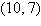b.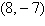c.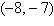d.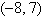e.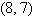2.

Find the distance between the two pointsand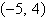.
 a. 9 b. 7 c. 4 d. 1 e. 2

3.

Determine which point lies on the graph of the equation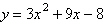.
 a. (0, –8) b. (1, –10) c. (0, –10) d. (1, –8) e. (2, –9)

4.

Use algebraic tests to check the following for symmetry with respect to the axes and the origin.a. Symmetric with respect to the y-axis. b. No symmetry. c. Symmetric with respect to the x-axis. d. Symmetric with respect to the origin.

5.

Use algebraic tests to check the following for symmetry with respect to the axes and the origin.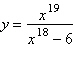a. No symmetry b. Symmetric with respect to the y-axis. c. Symmetric with respect to the x-axis. d. Symmetric with respect to the origin.

6.

You purchase a jet ski for \$10,000. The depreciated value, y, after x years is given by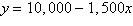. Sketch the graph of the equation given.
 a.d.b.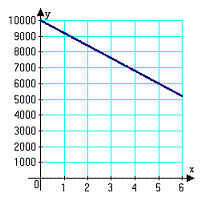e.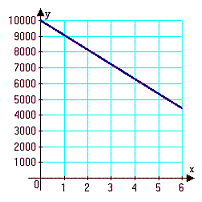c.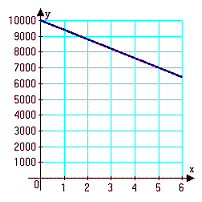7.

Does the table describe a function?
 Input value 10 30 10 20 40 Output value 2001 2002 2003 2004 2005
 a. yes b. no

8.

Does the table describe a function?
 Input value 2001 2002 2003 2004 2005 Output value 30 60 30 50 40
 a. yes b. no

9.

Which equation does not represent y as a function of x?
 a.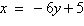b.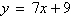c.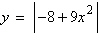d.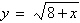e.10.

Evaluate the function at the specified value of the independent variable and simplify.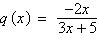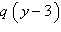a.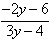b.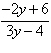c.d.e.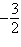11.

Find all real values of x such that f (x) = 0.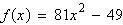a.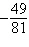b.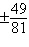c.d.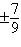e.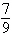12.

A rectangle is bounded by the x-axis and the semicircle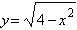(see figure). Write the area A of the rectangle as a function of x and determine the domain of the function.–2                                               2
 a.b.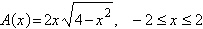c.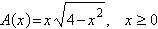d.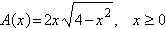e.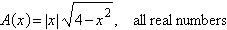13.

Use a graphing utility to graph the function and find the zeroes of the function.a.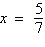b. no real zeroes c.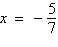d.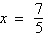e.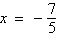14.

Use the position equation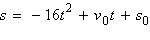to write a function that represents the situation and give the average velocity of the object from time t1 to time t2.
An object is thrown upward from a height of 174 feet at a velocity of 1 feet per second.
t1 = 1,     t2 = 5
 a.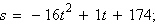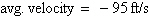b.c.d.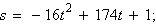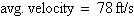e.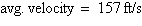15.

Find ( f + g)(x).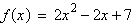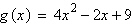a. ( f + g)(x) =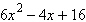b. ( f + g)(x) =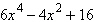c. ( f + g)(x) =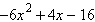d. ( f + g)(x) =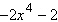e. ( f + g)(x) =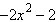16.

Evaluate the indicated function for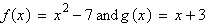.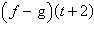a.b.c.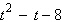d.e.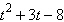17.

Find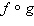.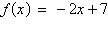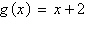a.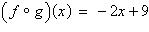b.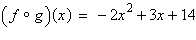c.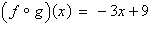d.e.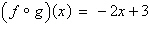18.

Find.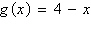a.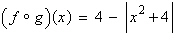b.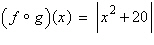c.d.e.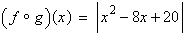19.

Find the inverse function of  f.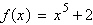a.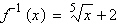b.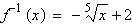c.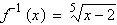d.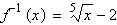e.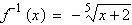20.

The sales tax on an item with a retail price of \$972 is \$77.76. Create a variational model that gives the retail price, y, in terms of the sales tax, x, and use it to determine the retail price of an item that has a sales tax of \$92.34.
 a. \$1156.24 b. \$1154.25 c. \$1098.56 d. \$1125.88 e. \$1166.48